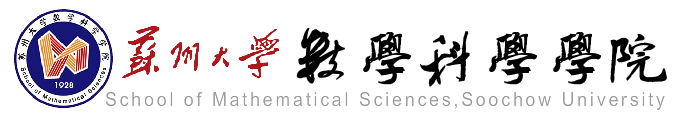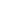科研动态最新资讯

• 天元讲堂（5.8）Carlos Vasquez：Linear response formula for the topological entropy at the time one map of a geodesic flow on a manifold of negative curvature
• 浏览量：116 发布人： 发布时间：2020-05-06
• 题目：Linear response formula for the topological entropy at the time one map of a geodesic flow on a manifold of  negative curvature

报告人：Carlos Vasquez (Pontificia Universidad Católica de Valparaíso)

时间：2020/5/8 10:00-11:00

线上讲座，Zoom会议信息见下

报告摘要：Let $f$ be the time one map of a geodesic flow on a manifold of constant negative curvature with $\mu$ its Liouville measure. Consider $f_t$, a $C^3$ family of diffeomorphisms with $f_0=f$. In this talk we discuss about the differentiability of the map $t\mapsto h_{\rm top}(f_t)$ at $t=0$, and we provide an explicit formula for its derivative.

This is a joint work with Pancho Valenzuela-Henr\'iquez and Radu Saghin from PUCV.

欢迎参加！

邀请人：动力系统与微分方程中心

腾讯会议信息：

会议时间：2020/5/08 10:00-11:30 https://us02web.zoom.us/j/3141591122?pwd=aWVGMGI2YkJQOXlEbk5IckRaRER2Zz09

会议 ID：314 159 1122

会议密码：123456